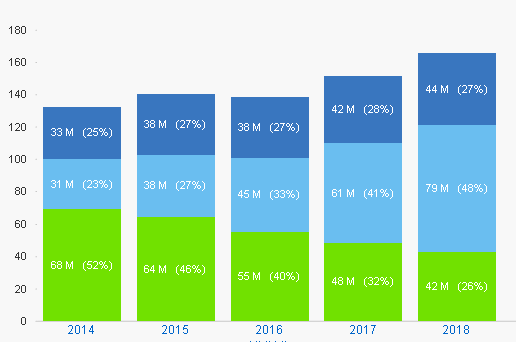# QlikView App Development

Discussion Board for collaboration related to QlikView App Development.

Announcements
Qlik Analytics Tour 2020 Online. Begins August 10th. Register Today
cancel
Showing results for
Did you mean:
HighlightedCreator

## How to insert a line break between 2 values in a bar chart ? (qlikview)

Hi the community,

Is it possible to insert a line break between the 2 values in a bar chart ?

Here I  use  the expression :

=dual(num(sum( {\$<Année=, Mois=, Média>}[ts Visites_supp2]/1000000),'#0 M') &
' (' & num(
(sum({\$<Année=, Mois=, Média>}[ts Visites_supp2]))/sum( {\$<Année=,Mois=,Média=>} TOTAL <Année>[ts Visites_supp2]),'##.0%') & ')',

num(sum( {\$<Année=, Mois=, Média>}[ts Visites_supp2]/1000000) )
+(sum({\$<Année=, Mois=, Média>}[ts Visites_supp2]))/sum( {\$<Année=,Mois=,Média=>} TOTAL <Année>[ts Visites_supp2]) )

I thought i could use "&chr(10)&", but it does not work.

Any idea ?Labels (1)
• ### bar chart expressions

2 Solutions

Accepted Solutions
HighlightedMVP & Luminary

## Re: How to insert a line break between 2 values in a bar chart ? (qlikview)

Usually it worked in this way. Did you try:

=dual(num(sum( {\$<Année=, Mois=, Média>}[ts Visites_supp2]/1000000),'#0 M') & chr(10) &
num(
(sum({\$<Année=, Mois=, Média>}[ts Visites_supp2]))/sum( {\$<Année=,Mois=,Média=>} TOTAL <Année>[ts Visites_supp2]),'(##.0%)'),

sum( {\$<Année=, Mois=, Média>}[ts Visites_supp2]/1000000)
+(sum({\$<Année=, Mois=, Média>}[ts Visites_supp2]))/sum( {\$<Année=,Mois=,Média=>} TOTAL <Année>[ts Visites_supp2])))

- Marcus

HighlightedMVP & Luminary

## Re: How to insert a line break between 2 values in a bar chart ? (qlikview)

Maybe this behaviour is release-dependent - for me in QV 11.2 it worked. To exclude any problems with your specific chart and any possible issues in regard to the settings there - create a new one with any small dimension maybe years and an expression like:

dual('a' & chr(10) & 'b' & chr(13) & 'c' & chr(10) & chr(13) & 'd', 1)

If this worked just add a second dimension and choose stacked bars and further settings step by step and you will see if any of these steps prevent the linebreak.

- Marcus

8 Replies
HighlightedMVP & Luminary

## Re: How to insert a line break between 2 values in a bar chart ? (qlikview)

Usually it worked in this way. Did you try:

=dual(num(sum( {\$<Année=, Mois=, Média>}[ts Visites_supp2]/1000000),'#0 M') & chr(10) &
num(
(sum({\$<Année=, Mois=, Média>}[ts Visites_supp2]))/sum( {\$<Année=,Mois=,Média=>} TOTAL <Année>[ts Visites_supp2]),'(##.0%)'),

sum( {\$<Année=, Mois=, Média>}[ts Visites_supp2]/1000000)
+(sum({\$<Année=, Mois=, Média>}[ts Visites_supp2]))/sum( {\$<Année=,Mois=,Média=>} TOTAL <Année>[ts Visites_supp2])))

- Marcus

HighlightedCreator

## Re: How to insert a line break between 2 values in a bar chart ? (qlikview)

Hello Marcus,

Yes i tried it but it does not work.

HighlightedMVP & Luminary

## Re: How to insert a line break between 2 values in a bar chart ? (qlikview)

What happens - no change, an error with the bars/values, wrong values or anything else? The number-format from tab Numbers is on expression?

HighlightedCreator

## Re: How to insert a line break between 2 values in a bar chart ? (qlikview)

the error message is "no data to display"HighlightedCreator

## Re: How to insert a line break between 2 values in a bar chart ? (qlikview)

Regarding the format, in the properties => 'default format' is selected

HighlightedMVP & Luminary

## Re: How to insert a line break between 2 values in a bar chart ? (qlikview)

Ok, remove the last bracket from the expression which is too much for a valid syntax. I was confused from all your brackets and didn't saw that the second sum() within the numeric-part was additionally wrapped with brackets - in this way they are superfluous. Are you sure that the 3 sum() parts are calculated in the wanted order?

- Marcus

HighlightedCreator

## Re: How to insert a line break between 2 values in a bar chart ? (qlikview)

Now the expression is

```=dual(num(sum( {\$<Année=, Mois=, Média>}[ts Visites_supp2]/1000000),'#0 M') & chr(10) &
num(
(sum({\$<Année=, Mois=, Média>}[ts Visites_supp2]))/sum( {\$<Année=,Mois=,Média=>} TOTAL <Année>[ts Visites_supp2]),'(##.0%)')
,

sum( {\$<Année=, Mois=, Média>}[ts Visites_supp2]/1000000)
+(sum({\$<Année=, Mois=, Média>}[ts Visites_supp2]))/sum( {\$<Année=,Mois=,Média=>} TOTAL <Année>[ts Visites_supp2]))```

and does not work.

I confirm you that the expression first calculates the Millions of visites in this format  '#0 M'.

Then it calculates the % of visits .

HighlightedMVP & Luminary

## Re: How to insert a line break between 2 values in a bar chart ? (qlikview)

Maybe this behaviour is release-dependent - for me in QV 11.2 it worked. To exclude any problems with your specific chart and any possible issues in regard to the settings there - create a new one with any small dimension maybe years and an expression like:

dual('a' & chr(10) & 'b' & chr(13) & 'c' & chr(10) & chr(13) & 'd', 1)

If this worked just add a second dimension and choose stacked bars and further settings step by step and you will see if any of these steps prevent the linebreak.

- Marcus